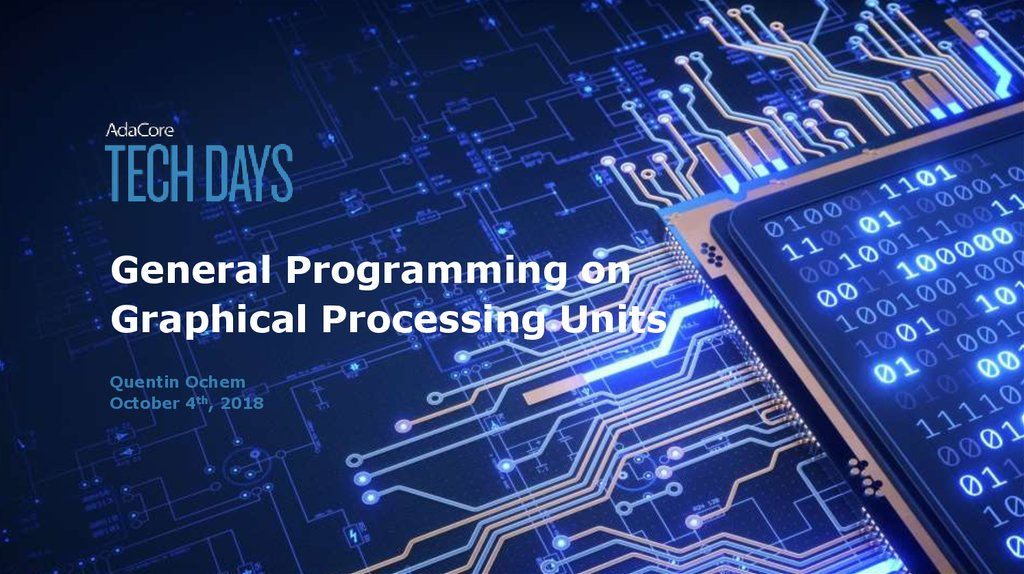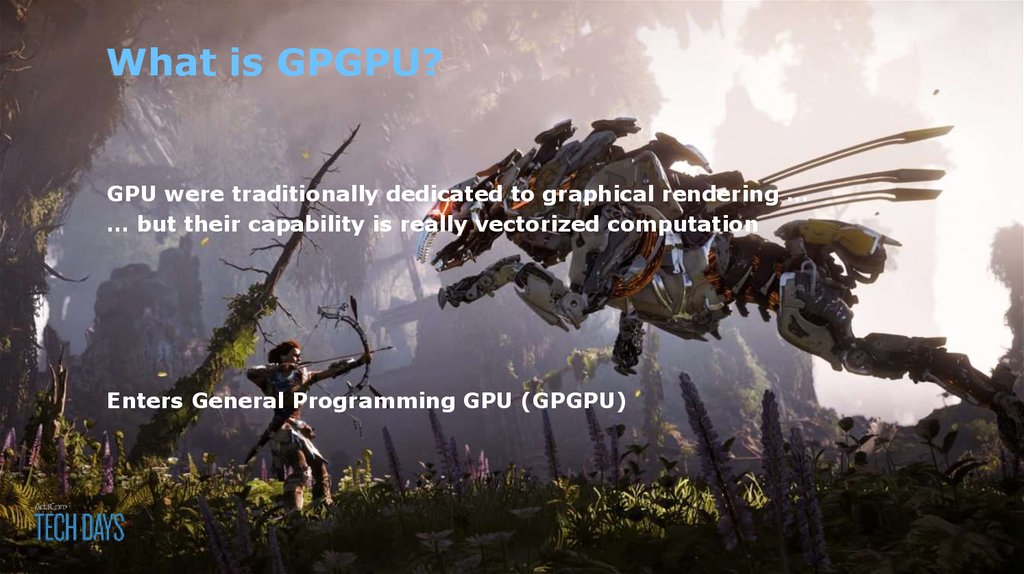# TechDays

## 2. General Programming on Graphical Processing Units

Quentin Ochem
October 4th, 2018

## 3. What is GPGPU?

GPU were traditionally dedicated to graphical rendering …
… but their capability is really vectorized computation
Enters General Programming GPU (GPGPU)

Debug?
Optimize data transfer?
core
core
core
core
core
core
core
core
core
Refactor parallel algorithms?
computations
Avoid data races?
How to optimize occupancy

- Signal processing
- Machine learning
- Monte-carlo simulation
- Trajectory prediction
- Cryptography
- Image processing
- Physical simulation
- … and much more!

## 7. Available Hardware

Desktop & Server
NVIDIA GeForce / Tesla / Quadro
Intel HD
Embedded
NVIDIA Tegra
ARM Mali
IMG Power VR
Freescale Vivante

## 9. Three options

Interfacing with existing libraries

## 10. Interfacing existing libraries

We could provide “thick” bindings to e.g. Ada.Numerics matrix
operations

CUDA – kernel-based language specific to NVIDIA
OpenCL – portable version of CUDA
OpenACC – integrated language marking parallel loops

## 12. CUDA Example (Device code)

procedure Test_Cuda
(A : out Float_Array; B, C : Float_Array)
with Export => True, Convention => C;
pragma CUDA_Kernel (Test_Cuda);
procedure Test_Cuda
(A : Float_Array; B, C : Float_Array)
is
begin
end Test_cuda;

## 13. CUDA Example (Host code)

A, B, C : Float_Array;
begin
-- initialization of B and C
-- CUDA specific setup
pragma CUDA_Kernel_Call (Grid’(1, 1, 1), Block’(8, 8, 8));
My_Kernel (A, B, C);
-- usage of A

## 14. OpenCL example

- Similar to CUDA in principle
- Requires more code on the host code (no call conventions)

## 15. OpenACC example (Device & Host)

OpenACC example (Device & Host)
procedure Test_OpenACC is
A, B, C : Float_Array;
begin
-- initialization of B and C
for I in A’Range loop
pragma Acc_Parallel;
A (I) := B (I) + C (I);
end loop;
end Test_OpenACC;

A, B, C : Float_Array;
begin
-- initialization of B and C
parallel for I in A’Range loop
A (I) := B (I) + C (I);
end loop;

## 17. Lots of other language considerations

- Identification of memory layout (per thread, per block, global)
- Reduction (ability to aggregate results through operators e.g.
sum or concatenation)
- Containers
- Mutual exclusion
-…

## 18. A word on SPARK

X_Size : 1000;
Y_Size : 10;
Data : array (1 .. X_Size * Y_Size) of Integer;
begin
for X in 1 .. X_Size loop
for Y in 1 .. Y_Size loop
Data (X + Y_Size * Y) := Compute (X, Y);
end loop;
end loop;
{X = 100, Y = 1}, X + Y * Y_Size = 100 + 10 = 110
{X = 10, Y = 10}, X + Y * Y_Size = 10 + 100 = 110

## 19. Next Steps

AdaCore spent 1 year to run various studies and experiments
Finalizing an OpenACC proof of concept on GCC
About to start an OpenCL proof of concept on CCG
If you want to give us feedback or register to try technology, contact us on
[email protected]